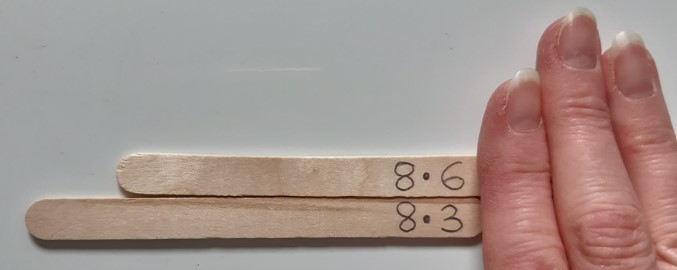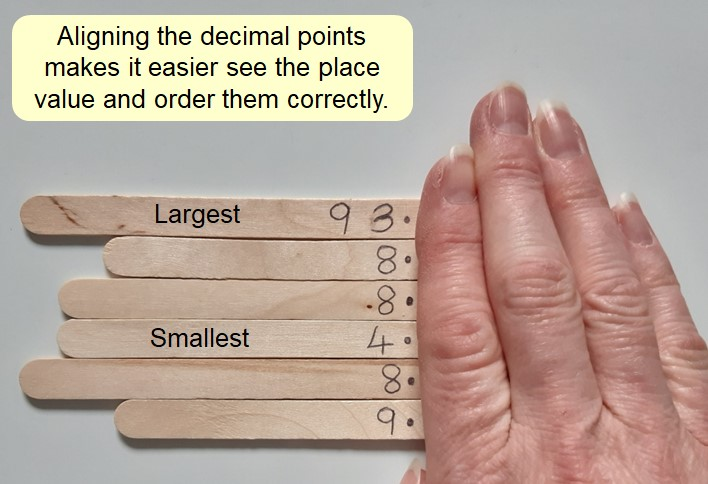## Comparing and ordering decimal numbers

When comparing decimal numbers, it is important to look carefully at the place value of each digit to prevent mistakes made by applying whole number thinking.

An easy and reliable way to do this is to find the decimal point and cover the digits to the right of it which form the decimal part of the number. This leaves just the whole number part visible, which can be compared to see which is larger. If both are equal, the next digit after the decimal point (the tenths) can be revealed and compared to see which is larger. If they are equal, the next digit (the hundredths) can be revealed and so on, until a difference between the digits allows the larger number to be identified.This process is even easier if the numbers are placed or written one beneath the other, with the decimal points aligned.The same process can be used when ordering decimals. All the numbers can be aligned and digits revealed one by one until the largest/smallest number is identified. This can then be removed and placed and positioned appropriately. The next largest/smallest number can then be identified, uncovering another digit if the visible digits are the same.